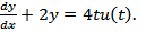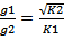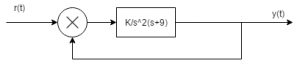Courses

Test: Time Response Of Second Order Systems - 1

10 Questions MCQ Test | Test: Time Response Of Second Order Systems - 1

Description
Attempt Test: Time Response Of Second Order Systems - 1 | 10 questions in 10 minutes | Mock test for Electrical Engineering (EE) preparation | Free important questions MCQ to study for Electrical Engineering (EE) Exam | Download free PDF with solutions
QUESTION: 1

Which of the following transfer function will have the greatest maximum overshoot?

Solution:

Explanation: Comparing the characteristic equation with the standard equation the value of the damping factor is calculated and the value for the option d is minimum hence the system will have the maximum overshoot .

QUESTION: 2

A system generated byThe ramp component in the forced response will be:

Solution:

Explanation:Laplace transforming
sY(s) + 2Y(s)=4/s2
Taking the inverse Laplace transform the forced term is 2t u(t).

QUESTION: 3

The system in originally critically damped if the gain is doubled the system will be :

Solution:

Explanation:hence due to this G lies between 0 and 1.

QUESTION: 4

Let c(t) be the unit step response of a system with transfer function K(s+a)/(s+K). If c(0+) = 2 and c(∞) = 10, then the values of a and K are respectively.

Solution:

Explanation: Applying initial value theorem which state that the initial value of the system is at time t =0 and this is used to find the value of K and final value theorem to find the value of a.

QUESTION: 5

The damping ratio and peak overshoot are measures of:

Solution:

Explanation: Speed of response is the speed at which the response takes the final value and this is determined by damping factor which reduces the oscillations and peak overshoot as the peak is less then the speed of response will be more.

QUESTION: 6

Find the type and order of the system given below:Solution:

Answer: Type = 2 which is the number of poles at the origin and order is the highest power of the characteristic equation.

QUESTION: 7

A system has a complex conjugate root pair of multiplicity two or more in its characteristic equation. The impulse response of the system will be:

Solution:

Explanation: Poles are the roots of the denominator of the transfer function and on imaginary axis makes the system stable but multiple poles makes the system unstable.

QUESTION: 8

The forward path transfer function is given by G(s) = 2/s(s+3). Obtain an expression for unit step response of the system.

Solution:

Explanation: C(s)/R(s) = s/(s2+3s+2)
C(s) = 1/s-2/s+1+1/s+2
c(t) = 1-2e-t+e+2t.

QUESTION: 9

Find the initial and final values of the following function:

F(s) = 12(s+1)/s(s+2)^2(s+3)

Solution:

Explanation: Using final and initial values theorem directly to find initial and final values but keeping in mind that final value theorem is applicable for stable systems only.

QUESTION: 10

The step response of the system is c(t) = 10+8e-t-4/8e-2t . The gain in time constant form of transfer function will be:

Solution:

Explanation: Differentiating the equation and getting the impulse response and then taking the inverse Laplace transform and converting the form into time constant form we get K = -7.5.Use Code STAYHOME200 and get INR 200 additional OFF Use Coupon Code

Track your progress, build streaks, highlight & save important lessons and more!

Similar ContentRelated tests